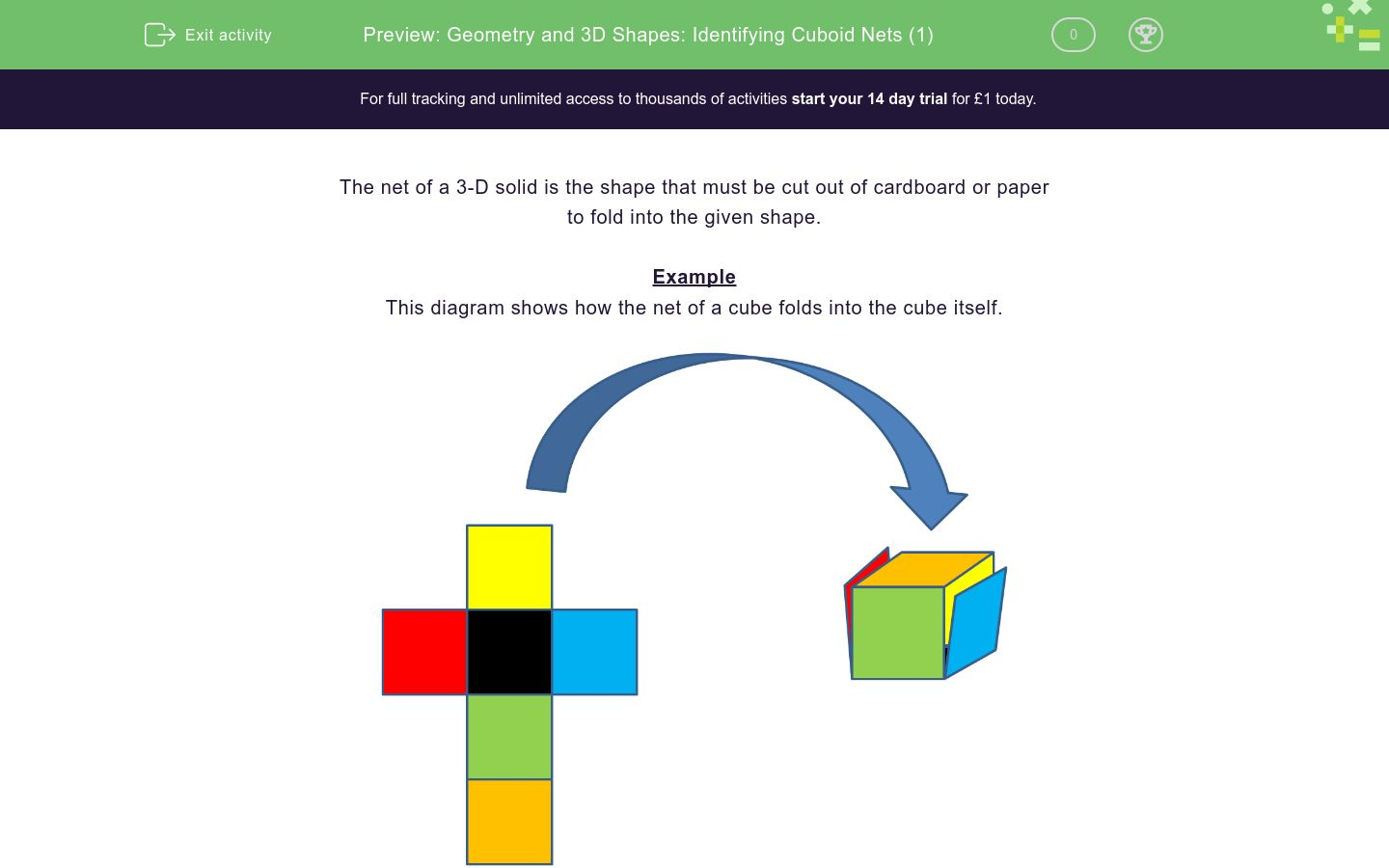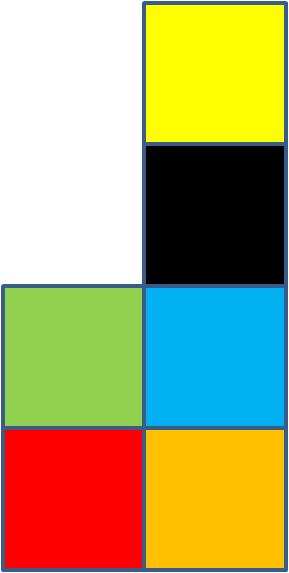# Geometry and 3D Shapes: Identifying Cuboid Nets (1)

In this worksheet, students answer 'True or false?' questions about cube nets.Key stage:  KS 2

Curriculum topic:  Geometry: Properties of Shapes

Curriculum subtopic:  Identify 3D Shapes

Difficulty level:### QUESTION 1 of 10

The net of a 3-D solid is the shape that must be cut out of cardboard or paper

to fold into the given shape.

Example

This diagram shows how the net of a cube folds into the cube itself.Remember that each cube will have the following...

A BASE, a TOP, a FRONT, a BACK, a LEFT and a RIGHT FACE

True or False?

This is a net for a cube.True

False

True or False?

This is a net for a cube.True

False

True or False?

This is a net for a cube.True

False

True or False?

This is a net for a cube.True

False

True or False?

This is a net for a cube.True

False

True or False?

This is a net for a cube.True

False

True or False?

This is a net for a cube.True

False

True or False?

This is a net for a cube.True

False

True or False?

This is a net for a cube.True

False

True or False?

This is a net for a cube.True

False

• Question 1

True or False?

This is a net for a cube.True
EDDIE SAYS
This is a net for a cube.
The black square would be the base of the cube. The red, yellow, blue and green squares would form the sides, and the orange square would be the top of the cube.
• Question 2

True or False?

This is a net for a cube.True
EDDIE SAYS
Again, the black square could form the base of the cube. The red, green, blue and yellow squares would form the sides and the orange cube would for the top.
• Question 3

True or False?

This is a net for a cube.False
EDDIE SAYS
This net has no square to become the base of the cube.
• Question 4

True or False?

This is a net for a cube.True
• Question 5

True or False?

This is a net for a cube.True
• Question 6

True or False?

This is a net for a cube.False
EDDIE SAYS
The squares in this net will overlap and will not form a proper cube.
• Question 7

True or False?

This is a net for a cube.False
EDDIE SAYS
Again, the squares in this net will not fold properly into a cube.
• Question 8

True or False?

This is a net for a cube.True
• Question 9

True or False?

This is a net for a cube.False
EDDIE SAYS
The three squares at the bottom of this net will overlap, so a proper cube can't be made.
• Question 10

True or False?

This is a net for a cube.